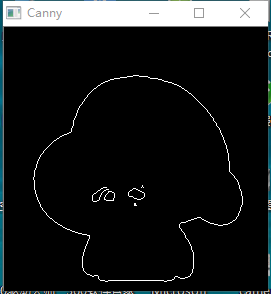0

2个回答

0

0openCV编程总结（2）-车道线检测之Bezier曲线3次拟合

2次三次4次Bezier曲线实践

1.什么是贝塞尔曲线？ 贝塞尔曲线所依据的最原始的数学公式，是早在1912年就广为人知的伯恩斯坦多项式。简单来说，伯恩斯坦多项式可以用来证明，在[ a, b ] 区间上所有的连续函数都可以用多项式来逼近，并且收敛性很强，也就是一致收敛。再简单点，就是一个连续函数，你可以将它写成若干个伯恩斯坦多项式相加的形式，并且，随着 n→∞，这个多项式将一致收敛到原函数，这个就是伯恩斯坦斯的逼近性质。

1.ValueAnimator通过AnimatorSet去play ValueAnimator valueAnimator = getBesselAnimator(tempImageView, rankWidth, rankHeight); AnimatorSet animatorSet = new AnimatorSet(); animatorSet.play(va
【算法+OpenCV】基于三次Bezier原理的曲线拟合算法C++与OpenCV实现
Bezier曲线拟合算法是一种相对较容易实现、且拟合的效果较好的算法。关于Bezier曲线原理，请参照（Bezier曲线原理），这里就不再做具体介绍了，我们使用的是Besier三次曲线拟合原理。下面主要介绍算法的实现过程。

Public Module BezierHelper     '''     ''' 获得二次贝塞尔曲线在某个时刻的位置     ''' B(t) = P0(1-t)^2+ 2P1t(1-t) + P2t^2     '''     ''' 起始点     ''' 二次贝塞尔曲线     ''' 时间t 0~1     Public Function GetPosition(p

C# 一种简单的贝塞尔拟合算法 前两天实现了一项功能，在一端进行书写，在另一端还原笔迹。由于两端的开发平台和绘图引擎不一致，书写端的笔迹很平滑，而另一端还原出来的笔迹为折线。为了使两端保持一致的效果，需要在还原端对笔迹进行贝塞尔拟合。本文将首先介绍贝塞尔曲线的基本原理及公式推导，然后提出一种简单的二次贝塞尔近似拟合算法，并用 C# 编程实现之。 贝塞尔曲线 相信大家都或多或少了解过贝塞尔曲线，...

bezier曲线拟合，opencv，车道线拟合

Matlab曲线拟合函数

webgl的贝塞尔曲线
BezierCurve webgl的贝塞尔曲线 webgl例子 https://www.khronos.org/webgl/wiki/Demo_Repository 参考 http://jsdo.it/Biske/A07Y http://jsdo.it/kimmy/Z9z6 [code=&quot;java&quot;] (function(d, w){ var FPS = 3...

python实现贝塞尔曲线拟合
python实现的贝塞尔曲线拟合，有测试数据
[TensorFlow深度学习入门]实战三·使用TensorFlow拟合曲线
[深度学习入门]题目二·使用TensorFlow拟合曲线 问题描述 拟合y= xx -2 x +3 + 0.1*(-1到1的随机值) 曲线 给定x范围（0，3） 问题分析 在上篇博客中，我们使用最简单的y=wx+b的模型成功拟合了一条直线，现在我们在进一步进行曲线的拟合。简单的y=wx+b模型已经无法满足我们的需求，需要利用更多的神经元来解决问题了。 生成数据 import nu...

%三次曲线规划函数： %x0,x1分别为规划起始位置和终止时刻位置，v0,v1分别为规划起始速度和终止时刻速度 %T为规划时长，t为求解时刻 function y=triple(x0,x1,v0,v1,T,t) a=(2*x0-2*x1+v0*T+v1*T)/T^3; b=(-3*x0+3*x1-2*v0*T-v1*T)/T^2; c=v0; d=x0; y=a*t^3+b*t^2

Size:=500 X := [25:50:Size] Y := 15 + 0.4 * X + 0.001 * X * X Y := Y + 40 * rand(|Y|) % 随机生成点集 gen_cross_contour_xld (Cross, Size - Y, X, 15, 0.785398) % 将坐标点变成十字架图标。 ///////////////////////////...

function [X,Y]=bezier(x,y) %用法： %bezier(x,y) % 生成n-1次贝塞尔曲线，其中x和y是n个点的坐标 %h=bezier(x,y) % 生成n-1次贝塞尔曲线并返回曲线句柄 %[X,Y]=bezier(x,y) % 返回n-1次贝塞尔曲线的坐标 %例子： %bezier([5,6,10,12],[0 5 -5 -2])

void myLMS_poly4(const std::vector<double> src_x, const std::vector<double> src_y, int size, std::vector<double>& dst_y) { double a, b, c, d, e; //Mat A = Mat_<double>(5, 5); //Mat B = Mat_<double>(5, 1); //Mat C = Mat_<double>(5, 1); Mat A(5, 5, CV_64